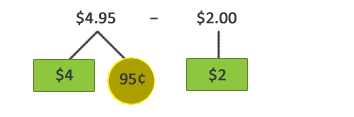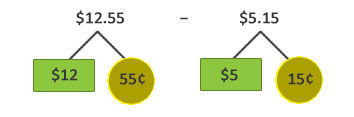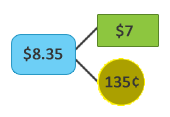## How to Subtract Money with Cents?

Practice Unlimited Questions

#### 1. Martin had \$4.95 at first. He bought a packet of gummies for \$2.00. How much money had he left?Step 1: Subtract the dollars.
\$4  −  \$2  =  \$2

\$2  +  95¢  =  \$2.95

#### 2. A T-shirt costs \$12.55. A bandana costs \$5.15 less. How much does the bandana cost?Step 1: Subtract the cents.
55¢  −  15¢  =  40¢

Step 2: Subtract the dollars.
\$12  −  \$5  =  \$7

Step 3: Add 40¢ to \$7.
\$7  +  40¢  =  \$7.40

The bandana costs \$7.40.

#### 3. \$8.35  −  \$3.75  =  ?Step 1: Regroup \$8.35.
\$8.35  =  \$7  +  135¢

Step 2: Subtract the cents.Step 3: Subtract the dollars.So,   \$8.35  −  \$3.75  =  \$4.60